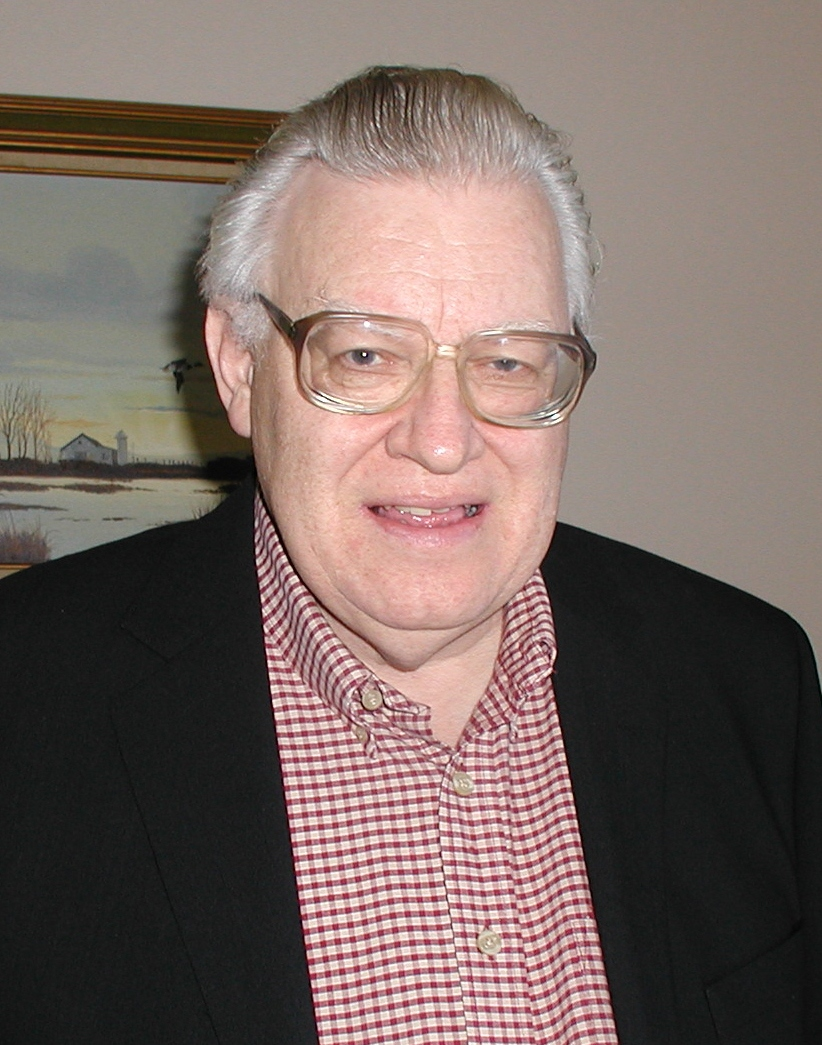Enter the content which will be displayed in sticky bar

# MemberGordon L. Ziegler
local time: 2021-07-28 18:42 (-07:00 DST)
Gordon L. Ziegler (Abstracts)
Titles
• [Updated 4 years ago]
Abstracts Details

The masses of charged leptons, the pion family, and the neutron family are here calculated, and the way is thereby paved for the calculation of the masses of every particle. This feat is not possible in the Quark Model, the Standard Model, the String Theory, or the Many-Dimensional Theory. It becomes possible only with the ?Electrino Hypothesis'; namely, that the ?fractional charges' used in modeling should be ?e/2, ?e/4, and ?e/8, and not the ?2e/3 and ?2e/3 used in the Quark Hypothesis. This Electrino Hypothesis is the basis for the far-reaching theory ?Electrino Fusion Model of Elementary Particles'. All that is used in this paper is the Electrino Hypothesis and algebra. All calculations are for either two-body problems or single-body problems. All particle bonds are assumed to be orbital bonds.

The derivation from first principles of the masses of three infinities of particles in this paper is a great test of the Electrino Fusion Model of Elementary Particles. Additional experimental tests for the Electrino Hypothesis are referenced in this paper also. It would take only a couple handfuls of newly discovered and measured particles to test the theory. These particles might be discovered soon at a new high-energy proton accelerator at CERN.

In the theory here set forth, electrinos (octons, quartons, semions, and unitons) are all trapped at or faster than the speed of light, and cannot go slower than the speed of light, so cannot be detected directly. Therefore the basis of the theory is more mathematical than physical, derived from first principles. Yet the model makes several physical predictions?the masses of two as yet unobserved charged leptons, two members of the pion family, and two members of the neutron family. They are all predicted to about three place accuracy.